﻿ Python Tkinter canvas shape editor: Draw and manipulate

# Python Tkinter canvas shape editor: Drawing and manipulating shapes

## Python Tkinter Canvas and Graphics: Exercise-13 with Solution

Write a Python program that allows the user to draw and manipulate geometric shapes (e.g., rectangle, circle) on the "Canvas" using Tkinter.

Sample Solution:

Python Code:

``````import tkinter as tk
class ShapeEditorApp:
def __init__(self, root):
self.root = root
self.root.title("Shape Editor")

# Create Canvas widget
self.canvas = tk.Canvas(root, bg="white")
self.canvas.pack(fill=tk.BOTH, expand=True)

# Initialize shape variables
self.current_shape = None
self.start_x = None
self.start_y = None
self.current_shape_item = None

# Create buttons
self.rect_button = tk.Button(root, text="Rectangle", command=self.create_rectangle)
self.circle_button = tk.Button(root, text="Circle", command=self.create_circle)
self.clear_button = tk.Button(root, text="Clear", command=self.clear_canvas)
self.rect_button.pack(side=tk.LEFT)
self.circle_button.pack(side=tk.LEFT)
self.clear_button.pack(side=tk.LEFT)

# Bind mouse events
self.canvas.bind("<Button-1>", self.start_draw)
self.canvas.bind("<B1-Motion>", self.draw_shape)
self.canvas.bind("<ButtonRelease-1>", self.stop_draw)

def create_rectangle(self):
self.current_shape = "rectangle"

def create_circle(self):
self.current_shape = "circle"

def start_draw(self, event):
self.start_x = event.x
self.start_y = event.y
if self.current_shape == "rectangle":
self.current_shape_item = self.canvas.create_rectangle(
self.start_x, self.start_y, self.start_x, self.start_y, outline="black"
)
elif self.current_shape == "circle":
self.current_shape_item = self.canvas.create_oval(
self.start_x, self.start_y, self.start_x, self.start_y, outline="black"
)

def draw_shape(self, event):
if self.current_shape_item:
x, y = event.x, event.y
self.canvas.coords(self.current_shape_item, self.start_x, self.start_y, x, y)

def stop_draw(self, event):
self.current_shape_item = None

def clear_canvas(self):
self.canvas.delete("all")

if __name__ == "__main__":
root = tk.Tk()
app = ShapeEditorApp(root)
root.mainloop()
```
```

Explanation:

In the exercise above -

1. Import the Tkinter library (tkinter).
2. Define the ShapeEditorApp class:
• The constructor init initializes the application and creates the main window (root).
• The main window's title is set to "Shape Editor."
3. Create a Canvas widget:
• A "Canvas" widget is created within the main window to serve as the drawing area. It's configured with a white background and expand to fill the available space.
4. Initialize shape-related variables:
• current_shape stores the currently selected shape ("rectangle" or "circle").
• start_x and start_y store the starting coordinates when drawing shapes.
• current_shape_item stores the currently drawn shape as a Canvas item.
5. Create buttons:
• Three buttons are created: "Rectangle," "Circle," and "Clear."
• The buttons are associated with functions (create_rectangle, create_circle, and clear_canvas) that will be called when the buttons are clicked.
• The buttons are packed (placed) on the main window in a horizontal layout using the pack method.
6. Bind mouse events:
• Three mouse events are bound to the Canvas widget:
• <Button-1> is bound to the start_draw method when the left mouse button is clicked.
• <B1-Motion> is bound to the draw_shape method when the left mouse button is moved.
• <ButtonRelease-1> is bound to the stop_draw method when the left mouse button is released.
7. Define functions for button actions:
• create_rectangle and create_circle set the current_shape variable to "rectangle" or "circle" when the respective buttons are clicked.
• start_draw initializes the drawing process by storing the starting coordinates and creating an initial shape (either a rectangle or a circle) at the starting point.
• draw_shape updates the shape's coordinates as the mouse is moved, allowing the user to draw the shape.
• stop_draw resets the current_shape_item to None when the drawing process is complete.
• clear_canvas deletes all items on the "Canvas" to clear the drawing.
8. Create the main application window:
• The root window is created using tk.Tk().
• An instance of the "ShapeEditorApp" class is created, which initializes the GUI and sets up the event handlers.
9. Start the Tkinter main loop.

Output:

```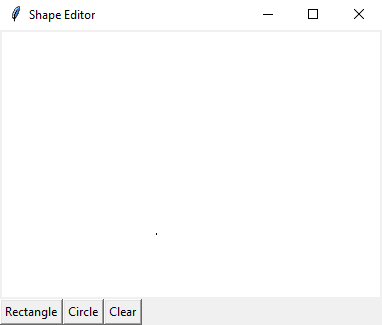```
```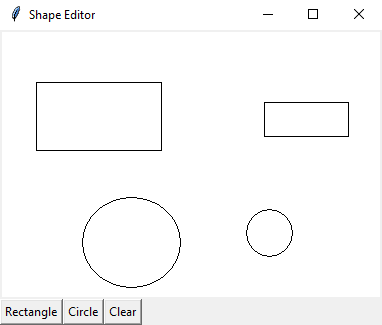```

Flowchart: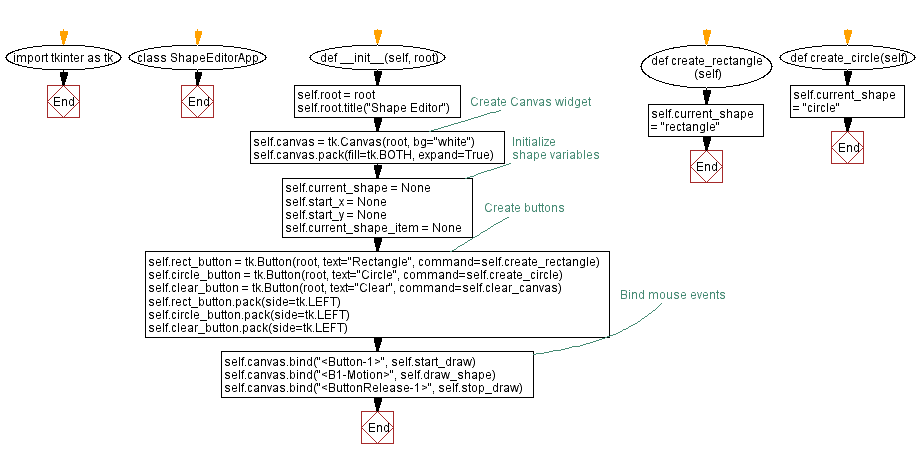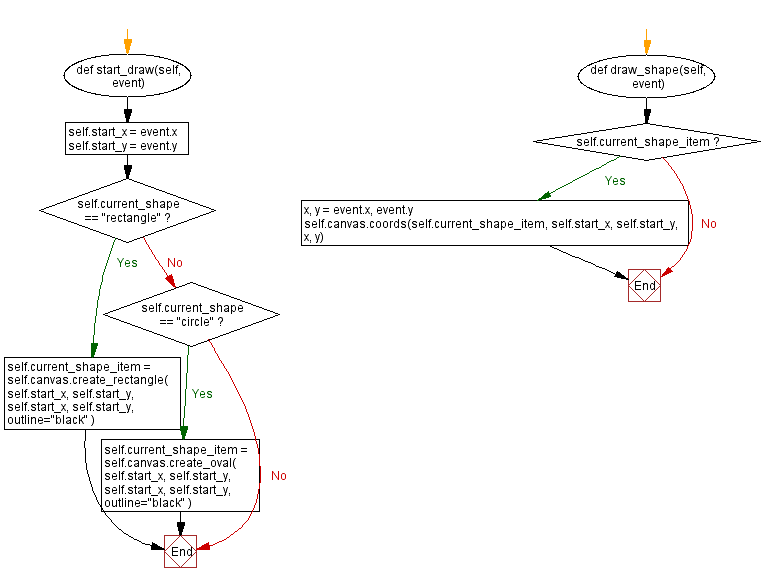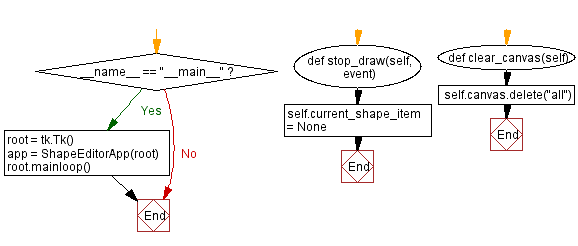Python Code Editor:

What is the difficulty level of this exercise?

Test your Programming skills with w3resource's quiz.

﻿

## Python: Tips of the Day

Memory Footprint Of An Object:

```import sys
```60